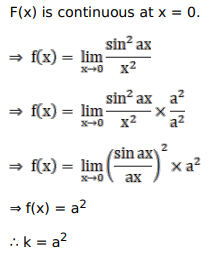# Solve this following

Question:

Mark $(\sqrt{)}$ against the correct answer in the following:

If the function $f(x)=\left\{\begin{array}{c}\frac{\sin ^{2} a x}{x^{2}}, \text { when } x \neq 0 \\ k, \text { when } x=0\end{array}\right.$ is continuous at $x=0$ then $k=?$

A. a

B. $\mathrm{a}^{2}$

C. $-2$

D. $-4$

Solution: